# 1. Introduction

Transcriptomic deconvolution in cancer and other heterogeneous tissues remains challenging. Available methods lack the ability to estimate both component-specific proportions and expression profiles for individual samples. We develop a three-component deconvolution model, DeMixT, for expression data from a mixture of cancerous tissues, infiltrating immune cells and tumor microenvironment. DeMixT is a software package that performs deconvolution on transcriptome data from a mixture of two or three components.

DeMixT is a frequentist-based method and fast in yielding accurate estimates of cell proportions and compart-ment-specific expression profiles for two-component three-component deconvolution problem. Our method promises to provide deeper insight into cancer biomarkers and assist in the development of novel prognostic markers and therapeutic strategies.

The function DeMixT is designed to finish the whole pipeline of deconvolution for two or three components. The newly added DeMixT_GS function is designed to estimates the proportions of mixed samples for each mixing component based on a new approach to select genes more effectively that utilizes profile likelihood. DeMixT_DE function is designed to estimate the proportions of all mixed samples for each mixing component based on the gene differential expressions to select genes. DeMixT_S2 function is designed to estimate the component-specific deconvolved expressions of individual mixed samples for a given set of genes.

# 2 Feature Description

The DeMixT R-package builds the transcriptomic deconvolution with a couple of novel features into R-based standard analysis pipeline through Bioconductor. DeMixT showed high accuracy and efficiency from our designed experiment. Hence, DeMixT can be considered as an important step towards linking tumor transcriptomic data with clinical outcomes.

Different from most previous computational deconvolution methods, DeMixT has integrated new features for the deconvolution with more than 2 components.

Joint estimation: jointly estimate component proportions and expression profiles for individual samples by requiring reference samples instead of reference genes; For the three-component deconvolution considering immune infiltration, it provides a comprehensive view of tumor-stroma-immune transcriptional dynamics, as compared to methods that address only immune subtypes within the immune component, in each tumor sample.

Efficient estimation: DeMixT adopts an approach of iterated conditional modes (ICM) to guarantee a rapid convergence to a local maximum. We also design a novel gene-set-based component merging approach to reduce the bias of proportion estimation for three-component deconvolutionthe.

Parallel computing: OpenMP enable parallel computing on single computer by taking advantage of the multiple cores shipped on modern CPUs. The ICM framework further enables parallel computing, which helps compensate for the expensive computing time used in the repeated numerical double integrations.

# 3. Installation

The DeMixT package is compatible with Windows, Linux and MacOS. The user can install it from Bioconductor:

if (!require("BiocManager", quietly = TRUE))
install.packages("BiocManager")

BiocManager::install("DeMixT")

For Linux and MacOS, the user can also install the latest DeMixT from GitHub:

if (!require("devtools", quietly = TRUE))
install.packages('devtools')

devtools::install_github("wwylab/DeMixT")

Check if DeMixT is installed successfully:

# load package
library(DeMixT)

Note: DeMixT relies on OpenMP for parallel computing. Starting from R 4.00, R no longer supports OpenMP on MacOS, meaning the user can only run DeMixT with one core on MacOS. We therefore recommend the users to mainly use Linux system for running DeMixT to take advantage of the multi-core parallel computation.

# 4. Functions

The following table shows the functions included in DeMixT.

DeMixT Deconvolution of tumor samples with two or three components.
DeMixT_GS Estimates the proportions of mixed samples for each mixing component based on a new approach to select genes that utilizes profile likelihood.
DeMixT_DE Estimates the proportions of mixed samples for each mixing component.
DeMixT_S2 Deconvolves expressions of each sample for unknown component.
Optimum_KernelC Call the C function used for parameter estimation in DeMixT.
DeMixT_Preprocessing Preprocessing functions before running DeMixT.

# 5. Methods

## 5.1 Model

Let $$Y_{ig}$$ be the observed expression levels of the raw measured data from clinically derived malignant tumor samples for gene $$g, g = 1, \cdots, G$$ and sample $$i, i = 1, \cdots, My$$. $$G$$ denotes the total number of probes/genes and $$My$$ denotes the number of samples. The observed expression levels for solid tumors can be modeled as a linear combination of raw expression levels from three components: ${Y_{ig}} = \pi _{1,i}N_{1,ig} + \pi _{2,i}N_{2,ig} + (1 - \pi_{1,i} - \pi _{2,i}){T_{ig}} \label{eq:1}$

Here $$N_{1,ig}$$, $$N_{2,ig}$$ and $${T_{ig}}$$ are the unobserved raw expression levels from each of the three components. We call the two components for which we require reference samples the $$N_1$$-component and the $$N_2$$-component. We call the unknown component the T-component. We let $$\pi_{1,i}$$ denote the proportion of the $$N_1$$-component, $$\pi_{2,i}$$ denote the proportion of the $$N_2$$-component, and $$1 - \pi_{1,i}-\pi_{2,i}$$ denote the proportion of the T-component. We assume that the mixing proportions of one specific sample remain the same across all genes.

Our model allows for one component to be unknown, and therefore does not require reference profiles from all components. A set of samples for $$N_{1,ig}$$ and $$N_{2,ig}$$, respectively, needs to be provided as input data. This three-component deconvolution model is applicable to the linear combination of any three components in any type of material. It can also be simplified to a two-component model, assuming there is just one $$N$$-component. For application in this paper, we consider tumor ($$T$$), stromal ($$N_1$$) and immune components ($$N_2$$) in an admixed sample ($$Y$$).

Following the convention that $$\log_2$$-transformed microarray gene expression data follow a normal distribution, we assume that the raw measures $$N_{1,ig} \sim LN({\mu _{{N_1}g}},\sigma _{{N_1}g}^2)$$, $$N_{2,ig} \sim LN({\mu _{{N_2}g}},\sigma _{{N_2}g}^2)$$ and $${T_{ig}} \sim LN({\mu _{Tg}}, \sigma _{Tg}^2)$$, where LN denotes a $$\log_2$$-normal distribution and $$\sigma _{{N_1}g}^2$$,$$\sigma _{{N_2}g}^2$$, $$\sigma _{Tg}^2$$ reflect the variations under $$\log_2$$-transformed data. Consequently, our model can be expressed as the convolution of the density function for three $$\log_2$$-normal distributions. Because there is no closed form of this convolution, we use numerical integration to evaluate the complete likelihood function (see the full likelihood in the Supplementary Materials in ).

## 5.2 The DeMixT algorithm for deconvolution

DeMixT estimates all distribution parameters and cellular proportions and reconstitutes the expression profiles for all three components for each gene and each sample. The estimation procedure (summarized in Figure 1b) has two main steps as follows.

1. Obtain a set of parameters $$\{\pi_{1,i}, \pi_{2,i}\}_{i=1}^{My}$$, $$\{\mu_T, \sigma_T\}_{g=1}^G$$ to maximize the complete likelihood function, for which $$\{\mu_{N_{1,g}}, \sigma_{N_{1,g}}, \mu_{N_{2,g}}, \sigma_{N_{2,g}}\}_{g=1}^G$$ were already estimated from the available unmatched samples of the $$N_1$$ and $$N_2$$ component tissues. (See further details in our paper.)

2. Reconstitute the expression profiles by searching each set of $$\{n_{1,ig}, n_{2,ig}\}$$ that maximizes the joint density of $$N_{1,ig}$$, $$N_{2,ig}$$ and $$T_{ig}$$. The value of $$t_{ig}$$ is solved as $${y_{ig}} - {{\hat \pi }_{1,i}}{n_{1,ig}} - {{\hat \pi }_{2,i}}{n_{2,ig}}$$.

These two steps can be separately implemented using the function DeMixT_DE or DeMixT_GS for the first step and DeMixT_S2 for the second, which are combined in the function DeMixT(Note: DeMixT_GS is the default function for first step).

Since version 1.8.2, DeMixT added simulated normal reference samples, i.e., spike-in, based on the observed normal reference samples. It has been shown to improve accuracy in proportion estimation for the scenario where a dataset consists of samples where true tumor proportions are skewed to the high end.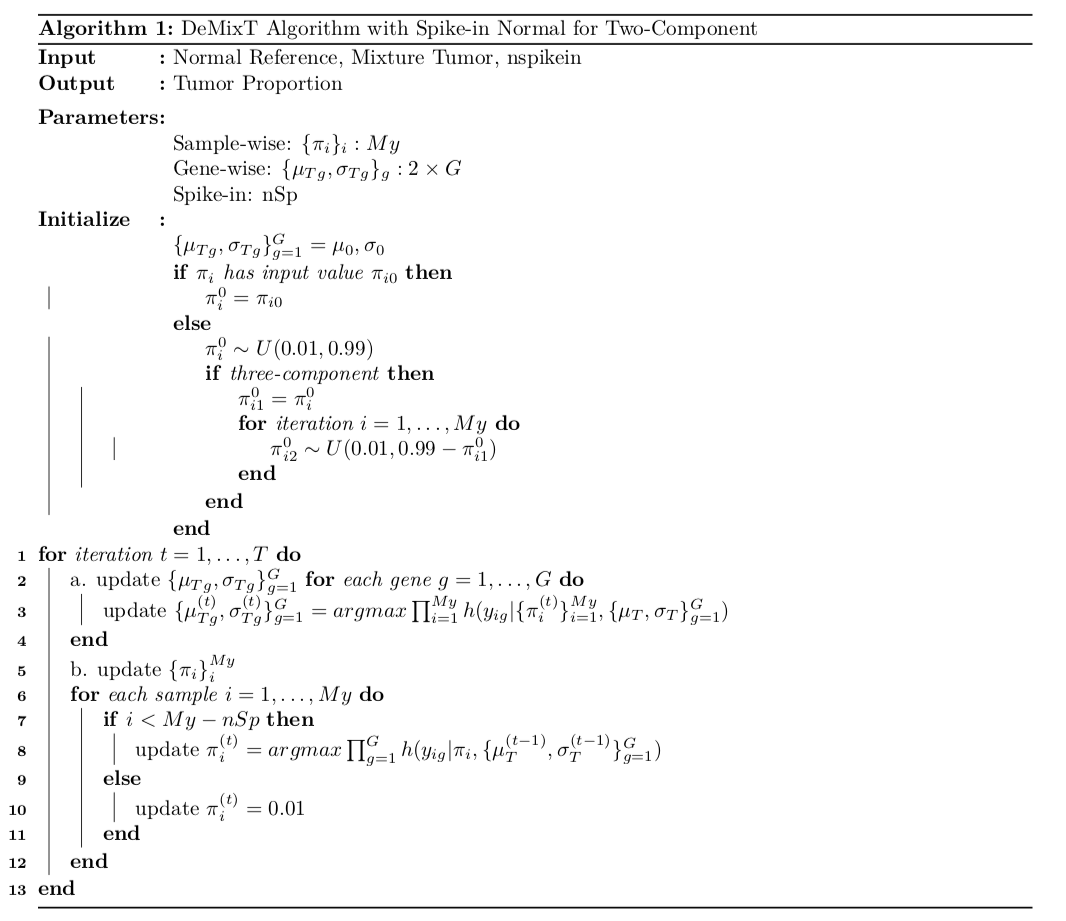# 6. Examples

## 6.1 Simulated two-component data

data("test.data.2comp")
# res.GS = DeMixT_GS(data.Y = test.data.2comp$data.Y, # data.N1 = test.data.2comp$data.N1,
#                     niter = 30, nbin = 50, nspikein = 50,
#                     if.filter = TRUE, ngene.Profile.selected = 150,
#                     mean.diff.in.CM = 0.25, ngene.selected.for.pi = 150,
#                     tol = 10^(-5))
load('Res_2comp/res.GS.RData')
head(t(res.GS$pi)) ## PiN1 PiT ## Sample 1 0.5955120 0.4044880 ## Sample 2 0.2759014 0.7240986 ## Sample 3 0.5401655 0.4598345 ## Sample 4 0.4497041 0.5502959 ## Sample 5 0.6516980 0.3483020 ## Sample 6 0.4365191 0.5634809 head(res.GS$gene.name)
##  "Gene 418" "Gene 452" "Gene 421" "Gene 112" "Gene 154" "Gene 143"
data("test.data.2comp")
# res.S2 <- DeMixT_S2(data.Y = test.data.2comp$data.Y, # data.N1 = test.data.2comp$data.N1,
#                     data.N2 = NULL,
#                     givenpi = c(t(res.S1$pi[-nrow(res.GS$pi),])), nbin = 50)
load('Res_2comp/res.S2.RData')
head(res.S2$decovExprT[,1:5],3) ## Sample 1 Sample 2 Sample 3 Sample 4 Sample 5 ## Gene 1 18.857446 60.727041 159.878946 92.031635 40.873852 ## Gene 2 2.322481 3.390938 2.406093 2.558962 2.438189 ## Gene 3 48.843631 208.166410 66.986239 38.107580 460.556751 head(res.S2$decovExprN1[,1:5],3)
##         Sample 1  Sample 2 Sample 3  Sample 4  Sample 5
## Gene 1  59.37087  71.80492  74.1755  73.55878  72.96267
## Gene 2 107.66874 131.20005 113.6376 120.35924 125.28224
## Gene 3 513.43184 669.79145 613.3042 491.09308 741.76507
head(res.S2$decovMu,3) ## MuN1 MuT ## Gene 1 6.166484 5.924321 ## Gene 2 6.677594 2.974551 ## Gene 3 9.329628 7.396647 head(res.S2$decovSigma,3)
##           SigmaN   SigmaT
## Gene 1 0.2222914 1.127726
## Gene 2 0.2319681 1.614169
## Gene 3 0.1881647 1.320477

## 6.2 Simulated two-component data

In the simulation,

## Simulate MuN and MuT for each gene
MuN <- rnorm(G, 7, 1.5)
MuT <- rnorm(G, 7, 1.5)
Mu <- cbind(MuN, MuT)
## Simulate SigmaN and SigmaT for each gene
SigmaN <- runif(n = G, min = 0.1, max = 0.8)
SigmaT <- runif(n = G, min = 0.1, max = 0.8)
## Simulate Tumor Proportion
PiT = truncdist::rtrunc(n = My,
spec = 'norm',
mean = 0.55,
sd = 0.2,
a = 0.25,
b = 0.95)

## Simulate Data
for(k in 1:G){

data.N1[k,] <- 2^rnorm(M1, MuN[k], SigmaN[k]); # normal reference

True.data.T[k,] <- 2^rnorm(My, MuT[k], SigmaT[k]);  # True Tumor

True.data.N1[k,] <- 2^rnorm(My, MuN[k], SigmaN[k]);  # True Normal

data.Y[k,] <- pi[1,]*True.data.N1[k,] + pi[2,]*True.data.T[k,] # Mixture Tumor

}

where $$\pi_i \in (0.25, 0.95)$$ is from truncated normal distribution. In general, the true distribution of tumor proportion does not follow a uniform distribution between $$[0,1]$$, but instead skewed to the upper part of the interval.

# ## DeMixT_DE without Spike-in Normal
# res.S1 = DeMixT_DE(data.Y = test.data.2comp$data.Y, # data.N1 = test.data.2comp$data.N1,
#                    niter = 30, nbin = 50, nspikein = 0,
#                    if.filter = TRUE,
#                    mean.diff.in.CM = 0.25, ngene.selected.for.pi = 150,
#                    tol = 10^(-5))
# ## DeMixT_DE with Spike-in Normal
# res.S1.SP = DeMixT_DE(data.Y = test.data.2comp$data.Y, # data.N1 = test.data.2comp$data.N1,
#                      niter = 30, nbin = 50, nspikein = 50,
#                      if.filter = TRUE,
#                      mean.diff.in.CM = 0.25, ngene.selected.for.pi = 150,
#                      tol = 10^(-5))
# ## DeMixT_GS with Spike-in Normal
# res.GS.SP = DeMixT_GS(data.Y = test.data.2comp$data.Y, # data.N1 = test.data.2comp$data.N1,
#                      niter = 30, nbin = 50, nspikein = 50,
#                      if.filter = TRUE, ngene.Profile.selected = 150,
#                      mean.diff.in.CM = 0.25, ngene.selected.for.pi = 150,
#                      tol = 10^(-5))
load('Res_2comp/res.GS.RData'); load('Res_2comp/res.GS.SP.RData'); 

This simulation was designed to compare previous DeMixT resutls with DeMixT spike-in results under both gene selection method.

res.2comp = as.data.frame(cbind(round(rep(t(test.data.2comp$pi[2,]),3),2), round(c(t(res.S1$pi[2,]),t(res.S1.SP$pi[2,]), t(res.GS.SP$pi[2,])),2),
rep(c('DE','DE-SP','GS-SP'), each = 100)), num = 1:2)
res.2comp$V1 <- as.numeric(as.character(res.2comp$V1))
res.2comp$V2 <- as.numeric(as.character(res.2comp$V2))
res.2comp$V3 = as.factor(res.2comp$V3)
names(res.2comp) = c('True.Proportion', 'Estimated.Proportion', 'Method')
## Plot
ggplot(res.2comp, aes(x=True.Proportion, y=Estimated.Proportion, group = Method, color=Method, shape=Method)) +
geom_point() +
geom_abline(intercept = 0, slope = 1, linetype = "dashed", color = "black", lwd = 0.5) +
xlim(0,1) + ylim(0,1)  +
scale_shape_manual(values=c(seq(1:3))) +
labs(x = 'True Proportion', y = 'Estimated Proportion')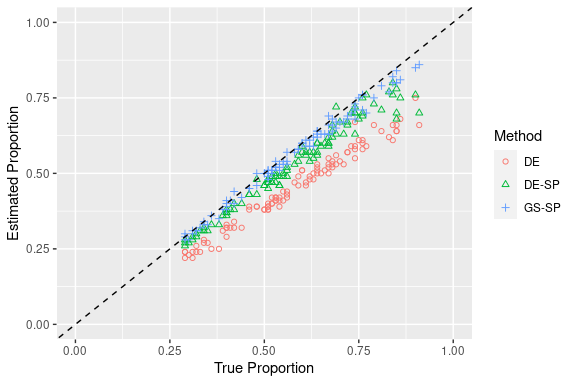## 6.3 Simulated three-component data

In this simulation,

G <- G1 + G2
## Simulate MuN1, MuN2 and MuT for each gene
MuN1 <- rnorm(G, 7, 1.5)
MuN2_1st <- MuN1[1:G1] + truncdist::rtrunc(n = 1,
spec = 'norm',
mean = 0,
sd = 1.5,
a = -0.1,
b = 0.1)
MuN2_2nd <- c()
for(l in (G1+1):G){
tmp <- MuN1[l] + truncdist::rtrunc(n = 1,
spec = 'norm',
mean = 0,
sd = 1.5,
a = 0.1,
b = 3)^rbinom(1, size=1, prob=0.5)
while(tmp <= 0) tmp <- MuN1[l] + truncdist::rtrunc(n = 1,
spec = 'norm',
mean = 0,
sd = 1.5,
a = 0.1,
b = 3)^rbinom(1, size=1, prob=0.5)
MuN2_2nd <- c(MuN2_2nd, tmp)
}
## Simulate SigmaN1, SigmaN2 and SigmaT for each gene
SigmaN1 <- runif(n = G, min = 0.1, max = 0.8)
SigmaN2 <- runif(n = G, min = 0.1, max = 0.8)
SigmaT <- runif(n = G, min = 0.1, max = 0.8)
## Simulate Tumor Proportion
pi <- matrix(0, 3, My)
pi[1,] <- runif(n = My, min = 0.01, max = 0.97)
for(j in 1:My){
pi[2, j] <- runif(n = 1, min = 0.01, max = 0.98 - pi[1,j])
pi[3, j] <- 1 - sum(pi[,j])
}
## Simulate Data
for(k in 1:G){

data.N1[k,] <- 2^rnorm(M1, MuN1[k], SigmaN1[k]); # normal reference 1

data.N2[k,] <- 2^rnorm(M2, MuN2[k], SigmaN2[k]); # normal reference 1

True.data.T[k,] <- 2^rnorm(My, MuT[k], SigmaT[k]);  # True Tumor

True.data.N1[k,] <- 2^rnorm(My, MuN1[k], SigmaN1[k]);  # True Normal 1

True.data.N2[k,] <- 2^rnorm(My, MuN2[k], SigmaN2[k]);  # True Normal 1

data.Y[k,] <- pi[1,]*True.data.N1[k,] + pi[2,]*True.data.N2[k,] +
pi[3,]*True.data.T[k,] # Mixture Tumor

}

where $$G1$$ is the number of genes that $$\mu_{N1}$$ is close to $$\mu_{N2}$$.

data("test.data.3comp")
# res.S1 <- DeMixT_DE(data.Y = test.data.3comp$data.Y, data.N1 = test.data.3comp$data.N1,
#                    data.N2 = test.data.3comp$data.N2, if.filter = TRUE) load('Res_3comp/res.S1.RData');  res.3comp= as.data.frame(cbind(round(t(matrix(t(test.data.3comp$pi), nrow = 1)),2),
round(t(matrix(t(res.S1$pi), nrow = 1)),2), rep(c('N1','N2','T'), each = 20))) res.3comp$V1 <- as.numeric(as.character(res.3comp$V1)) res.3comp$V2 <- as.numeric(as.character(res.3comp$V2)) res.3comp$V3 = as.factor(res.3comp$V3) names(res.3comp) = c('True.Proportion', 'Estimated.Proportion', 'Component') ## Plot ggplot(res.3comp, aes(x=True.Proportion, y=Estimated.Proportion, group = Component, color=Component, shape=Component)) + geom_point() + geom_abline(intercept = 0, slope = 1, linetype = "dashed", color = "black", lwd = 0.5) + xlim(0,1) + ylim(0,1) + scale_shape_manual(values=c(seq(1:3))) + labs(x = 'True Proportion', y = 'Estimated Proportion')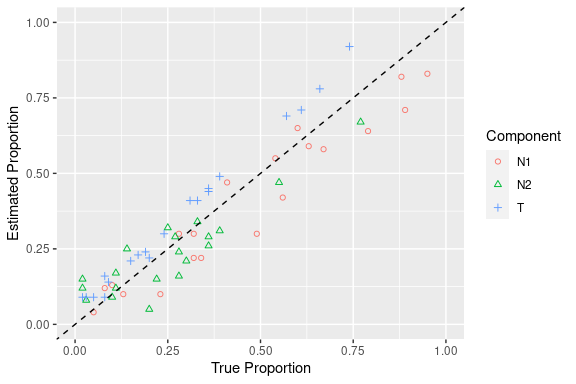## 6.4 Real data: PRAD in TCGA dataset ### 6.4.1 Preprocessing For the deconvolution of real data with DeMixT, the user may apply the following preprocessing steps first. Here, we use the PRAD (prostate adenocarcinoma) from TCGA as an example. 1. (Optional) Remove suspicious samples by hierarchical clustering It is possible that the some of the tumor and normal samples are mislabelled. We use the function # hc_labels <- detect_suspicious_sample_by_hierarchical_clustering_2comp(count.matrix, normal.id, tumor.id) to visually inspect the separation of tumor and normal samples based on the hierarchical clustering of their expressions, in which count.matrix is the raw count matrix; normal.id and tumor.id are the vectors of normal and tumor sample ids, respectively. Generally, one cluster contains tumor samples and the other contains normal samples. Any samples that are clustered outside of its own group label, e.g., tumor samples clustered within the normal sample cluster or normal samples in the tumor cluster, are considered as mislabelled samples and filtered out.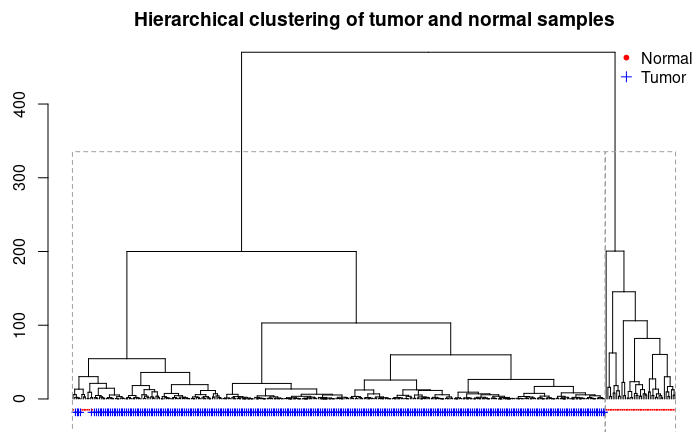count.matrix, normal.id and tumor.id are updated by # normal.id <- setdiff(normal.id, names(hc_labels$cluster[hc_labels$cluster == 1])) # tumor.id <- setdiff(tumor.id, names(hc_labels$cluster[hc_labels$cluster == 2])) # count.matrix <- count.matrix[, c(normal.id, tumor.id)] 1. Select genes with small variation in gene expression across samples In this step, we select a subset of ~9000 genes from the original gene set (>50,000) before running DeMixT with the GS (Gene Selection) method so that our model-based gene selection maintains good statistical properties. We use the function # plot_sd(count.matrix, normal.id, tumor.id) to visualize the distribution of standard deviation of log2 expressions space of normal and tumor samples,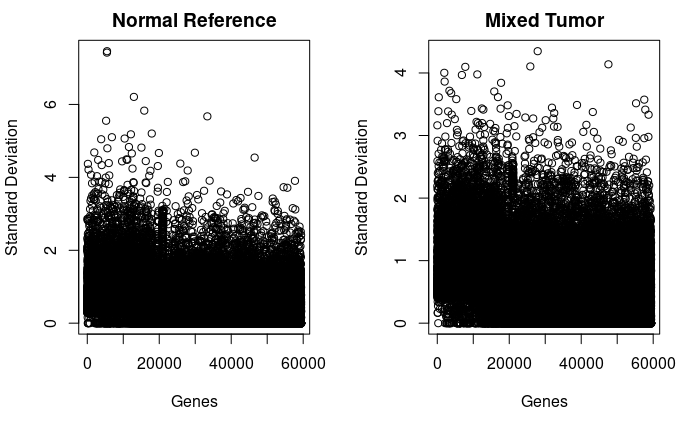and use the function # num_gene_remaining_different_cutoffs <- subset_sd_gene_remaining(count.matrix, normal.id, tumor.id, cutoff_normal_range = c(0.1, 1.0), cutoff_tumor_range = c(0, 2.5), cutoff_step = 0.1) to find he a range of variance in genes from normal samples (cutoff_normal_range) and from tumor samples (cutoff_tumor_range) which results in roughly 9,000 genes. We then use the function # count.matrix <- subset_sd(count.matrix, normal.id, tumor.id, cutoff_normal = cutoff_normal_range, cutoff_tumor = cutoff_tumor_range) to update the count.matrix such that it only includes the selected genes. 1. Scale normalization We apply a scale normalization at the 75th percentile across all the tumor and normal samples using the function # count.matrix <- scale_normalization_75th_percentile(count.matrix) to adjust the expression levels in the samples. Note: The user may also use the function # count.matrix <- DeMixT_preprocessing(count.matrix, normal.id, tumor.id, cutoff_normal_range = c(0.1, 1.0), cutoff_tumor_range = c(0, 2.5), cutoff_step = 0.1) to perform the preprocessing steps of 2) and 3) in one go. 1. (Optional) Batch effect correction for tumor samples from different batches by ComBat If the tumor samples are from different batches, we recommend the user to inspect the batch effect using the function before running DeMixT # plot_dim(count.matrix.tumor, labels, legend.position = 'bottomleft', legend.cex = 1.2) This function will generate a PCA plot, in which the samples are colored by the labels indicating different batches of tumor samples, as well as normal samples. If there is a clear separation between different batches of tumor samples, there is likely batch effects. We use the function # count.matrix.tumor <- batch_correction(count.matrix.tumor, labels) to reduce this effect, where count.matrix.tumor is the raw count matrix only from tumor samples and labels is the factor of tumor batches. The user may choose other batch effect correction methods at this step. The user may inspect the batch effect again after the above step using the mentioned function # plot_dim(count.matrix.tumor, labels, legend.position = 'bottomleft', legend.cex = 1.2) ### 6.4.2 Deconvolution using DeMixT To optimize the DeMixT parameter setting for the input data, we recommend testing an array of combinations of the number of spike-ins and the number of selected genes. The number of CPU cores used by the DeMixT function for parallel computing is specified by the parameter nthread. By default (such as in the code block below), nthread = total_number_of_cores_on_the_machine - 1. The user can change nthread to a number between 0 and the total number of cores on the machine. ## Because of the random initial values and the spike-in samples within the DeMixT function, ## we would like to remind the user to set seeds to keep track. This seed setting will be ## internalized in DeMixT in the next update. # set.seed(1234) # data.Y = SummarizedExperiment(assays = list(counts = count.matrix[, tumor.id])) # data.N1 <- SummarizedExperiment(assays = list(counts = count.matrix[, normal.id])) ## In practice, we set the maximum number of spike-in as min(n/3, 200), ## where n is the number of samples. # nspikesin_list = c(0, 50, 100, 150) ## One may set a wider range than provided below for studies other than TCGA. # ngene.selected_list = c(500, 1000, 1500, 2500) #for(nspikesin in nspikesin_list){ # for(ngene.selected in ngene.selected_list){ # name = paste("PRAD_demixt_GS_res_nspikesin", nspikesin, "ngene.selected", # ngene.selected, sep = "_"); # name = paste(name, ".RData", sep = ""); # res = DeMixT(data.Y = data.Y, # data.N1 = data.N1, # ngene.selected.for.pi = ngene.selected, # ngene.Profile.selected = ngene.selected, # filter.sd = 0.7, # same upper bound of gene expression standard deviation # # for normal reference. i.e., preprocessed_data$sd_cutoff_normal
#                     gene.selection.method = "GS",
#                     nspikein = nspikesin)
#        save(res, file = name)
#    }
#}

We suggest selecting the optimal parameter combination that produces the largest average correlation of estimated tumor propotions with those produced by other combinations. The location of the mode of the Pi estimation may also be considered. The mode located too high or too low may suggest biased estimation.

Instead of selecting using the parameter combination with the highest correlation, one can also select the parameter combination that produces estimated tumor proportions that are most biologically meaningful.

A comprehensive tutorial of using DeMixT for real data deconvolution can be found at https://wwylab.github.io/DeMixT/tutorial.html.

## 6.5 Deconvolution using normal reference samples from GTEx

We conducted experiments across cancer types to evaluate the impact of technical artifacts such as batch effects to the proportion estimation when using a different cohort. We applied GTEx expression data from normal prostate samples as the normal reference to deconvolute the TCGA prostate cancer samples, where normal tissues were selected without significant pathology. The estimated proportions showed a reasonable correlation (Spearman correlation coefficient = 0.65) with those generated using TCGA normal prostate samples as the normal reference.

## Deconvolute TCGA prostate cancer samples from GTEx normal samples
niter = 50, nbin = 50, nspikein = 49, filter.sd = 0.6,
if.filter = TRUE, ngene.Profile.selected = 1500,
mean.diff.in.CM = 0.25, ngene.selected.for.pi = 1500,
tol = 10^(-5))
## Deconvolute TCGA prostate cancer samples from TCGA normal samples
niter = 50, nbin = 50, nspikein = 49, filter.sd = 0.6,
if.filter = TRUE, ngene.Profile.selected = 1500,
mean.diff.in.CM = 0.25, ngene.selected.for.pi = 1500,
tol = 10^(-5))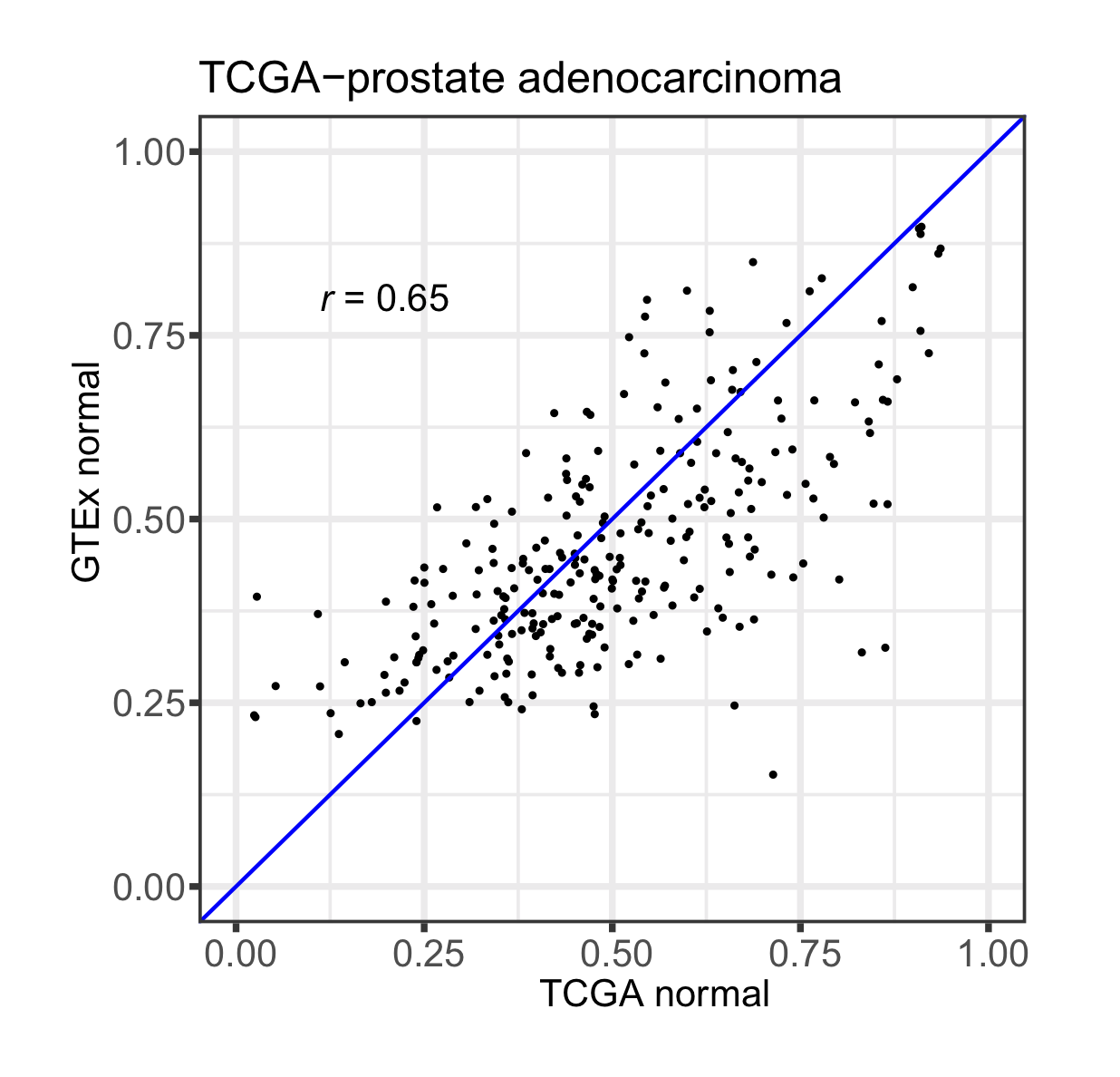# 7. Reference

. Wang, Z. et al. Transcriptome Deconvolution of Heterogeneous Tumor Samples with Immune Infiltration. iScience 9, 451–460 (2018).

# 8. Session Info

sessionInfo(package = "DeMixT")
## R version 4.2.1 (2022-06-23)
## Platform: x86_64-pc-linux-gnu (64-bit)
## Running under: Ubuntu 20.04.5 LTS
##
## Matrix products: default
## BLAS:   /home/biocbuild/bbs-3.16-bioc/R/lib/libRblas.so
## LAPACK: /home/biocbuild/bbs-3.16-bioc/R/lib/libRlapack.so
##
## locale:
##   LC_CTYPE=en_US.UTF-8       LC_NUMERIC=C
##   LC_TIME=en_GB              LC_COLLATE=C
##   LC_MONETARY=en_US.UTF-8    LC_MESSAGES=en_US.UTF-8
##   LC_PAPER=en_US.UTF-8       LC_NAME=C
##  LC_MEASUREMENT=en_US.UTF-8 LC_IDENTIFICATION=C
##
## attached base packages:
## character(0)
##
## other attached packages:
##  DeMixT_1.14.0
##
## loaded via a namespace (and not attached):
##    colorspace_2.0-3            bsseq_1.34.0
##    rjson_0.2.21                XVector_0.38.0
##    GenomicRanges_1.50.0        base64enc_0.1-3
##    farver_2.1.1                stats_4.2.1
##    bit64_4.0.5                 AnnotationDbi_1.60.0
##   fansi_1.0.3                 codetools_0.2-18
##   splines_4.2.1               R.methodsS3_1.8.2
##   sparseMatrixStats_1.10.0    mnormt_2.1.1
##   cachem_1.0.6                knitr_1.40
##   jsonlite_1.8.3              Rsamtools_2.14.0
##   annotate_1.76.0             base_4.2.1
##   png_0.1-7                   R.oo_1.25.0
##   DSS_2.46.0                  HDF5Array_1.26.0
##   compiler_4.2.1              httr_1.4.4
##   assertthat_0.2.1            Matrix_1.5-1
##   fastmap_1.1.0               limma_3.54.0
##   cli_3.4.1                   htmltools_0.5.3
##   tools_4.2.1                 gtable_0.3.1
##   glue_1.6.2                  GenomeInfoDbData_1.2.9
##   dplyr_1.0.10                grDevices_4.2.1
##   Rcpp_1.0.9                  Biobase_2.58.0
##   jquerylib_0.1.4             vctrs_0.5.0
##   Biostrings_2.66.0           rhdf5filters_1.10.0
##   nlme_3.1-160                rtracklayer_1.58.0
##   DelayedMatrixStats_1.20.0   psych_2.2.9
##   xfun_0.34                   stringr_1.4.1
##   lifecycle_1.0.3             restfulr_0.0.15
##   gtools_3.9.3                XML_3.99-0.12
##   dendextend_1.16.0           edgeR_3.40.0
##   zlibbioc_1.44.0             scales_1.2.1
##   BSgenome_1.66.0             graphics_4.2.1
##   MatrixGenerics_1.10.0       parallel_4.2.1
##   SummarizedExperiment_1.28.0 rhdf5_2.42.0
##   utils_4.2.1                 yaml_2.3.6
##   memoise_2.0.1               gridExtra_2.3
##   ggplot2_3.3.6               sass_0.4.2
##   datasets_4.2.1              stringi_1.7.8
##   RSQLite_2.2.18              highr_0.9
##   genefilter_1.80.0           S4Vectors_0.36.0
##   BiocIO_1.8.0                permute_0.9-7
##   BiocGenerics_0.44.0         BiocParallel_1.32.0
##   GenomeInfoDb_1.34.0         rlang_1.0.6
##   pkgconfig_2.0.3             matrixStats_0.62.0
##   bitops_1.0-7                evaluate_0.17
##   lattice_0.20-45             Rhdf5lib_1.20.0
##   GenomicAlignments_1.34.0    labeling_0.4.2
##   bit_4.0.4                   tidyselect_1.2.0
##   magrittr_2.0.3              R6_2.5.1
##   IRanges_2.32.0              generics_0.1.3
##   DelayedArray_0.24.0         DBI_1.1.3
##  pillar_1.8.1                withr_2.5.0
##  mgcv_1.8-41                 survival_3.4-0
##  KEGGREST_1.38.0             RCurl_1.98-1.9
##  tibble_3.1.8                crayon_1.5.2
##  KernSmooth_2.23-20          utf8_1.2.2
##  rmarkdown_2.17              viridis_0.6.2
##  locfit_1.5-9.6              grid_4.2.1
##  sva_3.46.0                  data.table_1.14.4
##  blob_1.2.3                  methods_4.2.1
##  matrixcalc_1.0-6            digest_0.6.30
##  xtable_1.8-4                R.utils_2.12.1
##  stats4_4.2.1                munsell_0.5.0
##  viridisLite_0.4.1           bslib_0.4.0